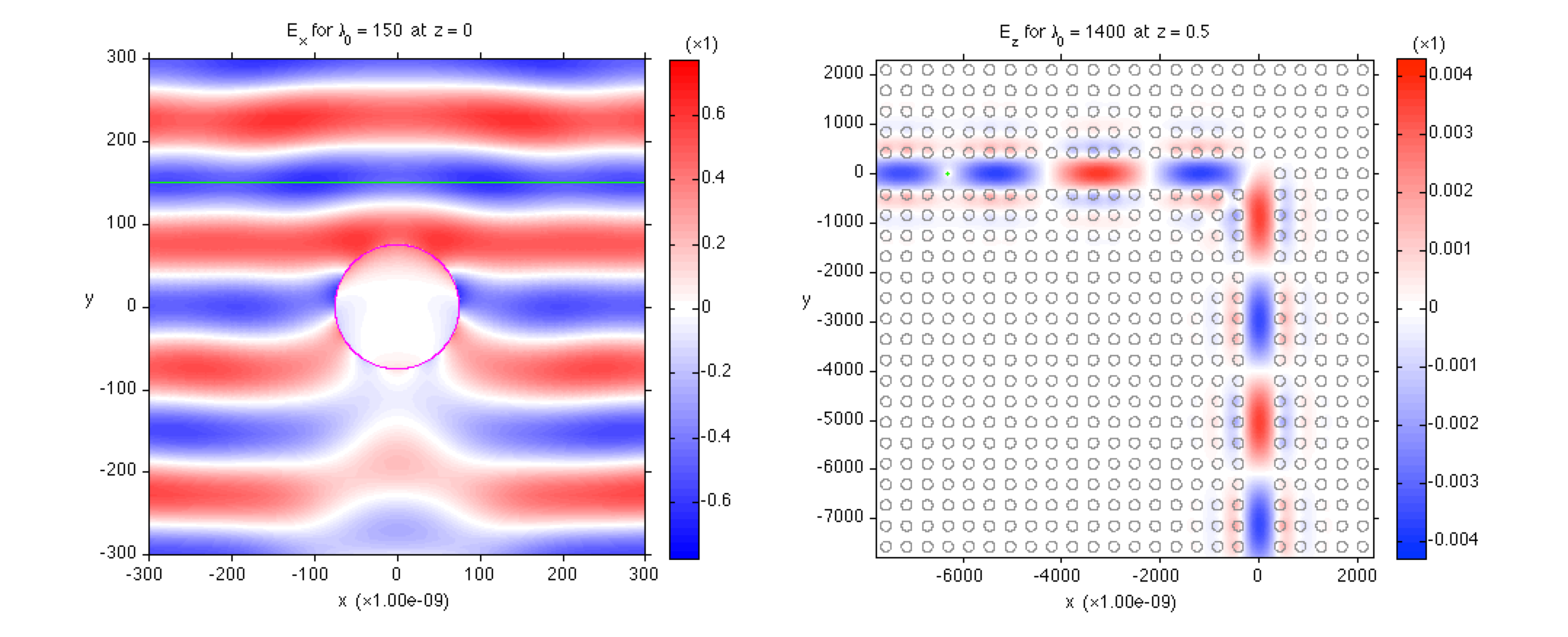# EE256: Numerical Electromagneics (Stanford University, Spring 2014)You probably have learned Maxwell's equations, which are the following four coupled partial differential equations (PDEs):

$\nabla \cdot \mathbf{D} = \rho_f\;,\;\nabla \cdot \mathbf{B} = 0,\\ \nabla \times \mathbf{E} = -\frac{\partial\mathbf{B}}{\partial t}\;,\;\nabla \times \mathbf{H} = \mathbf{J}_f - \frac{\partial\mathbf{D}}{\partial t}.$

They are governing equations behind many of the modern technologies, such as radio and TV broadcasting, satellite and optical communication, radar, lasers, and solar cells. However, for realistic systems beyond simple textbook examples, these equations are in general difficult to solve. Take EE256 and you will

• learn to solve Maxwell's equations for realistic systems;

• learn to conceive the propagation of electromagnetic waves through complicated media; and

• form a better than ever understanding of Maxwell's equations.

Already a user of some numerical solver of Maxwell's equations? Take EE256 and you will

• gain a better understanding of the numerical solver; and

• understand the proper way of setting up your simulation.

## Topics to Be Covered

• Finite-difference time-domain (FDTD) method of solving Maxwell's equations

• Finite-difference frequency-domain (FDFD) method of solving Maxwell's equations

• TE and TM wave decomposition

• Perfectly matched layer (PML) absorbing boundaries for simulating infinite space

• Total-field/scattered-field (TF/SF) method for analyzing scattering events

• Numerical stability and numerical dispersion

• Simulating dispersive materials

• Advanced topics: fundamental origin of numerical error, limitations of PML, waveguide mode solver, tips on visualization, and more!

## Key Differences from Previous Years

• The FDTD method and the FDFD method. For the first time, the two different methods of solving Maxwell's equations will be taught at the same time. By learning the different pros and cons of the FDTD and FDFD methods, you will understand that no single numerical method is perfect for all problems.

• Smaller, more frequent programming assignments. In the previous years, big programming assignments given every two weeks were major frustrations for the students. This year, we will divide programming assignments into smaller, more manageable weekly assignments. We will also provide template source codes that the students can start with.

• No midterm exam. Homework assignments and final projects only.